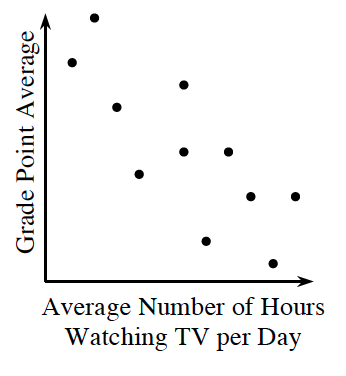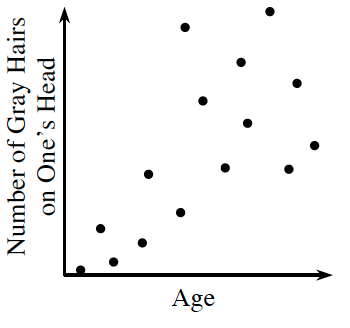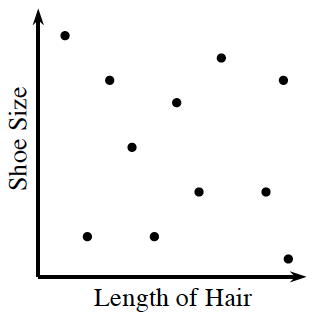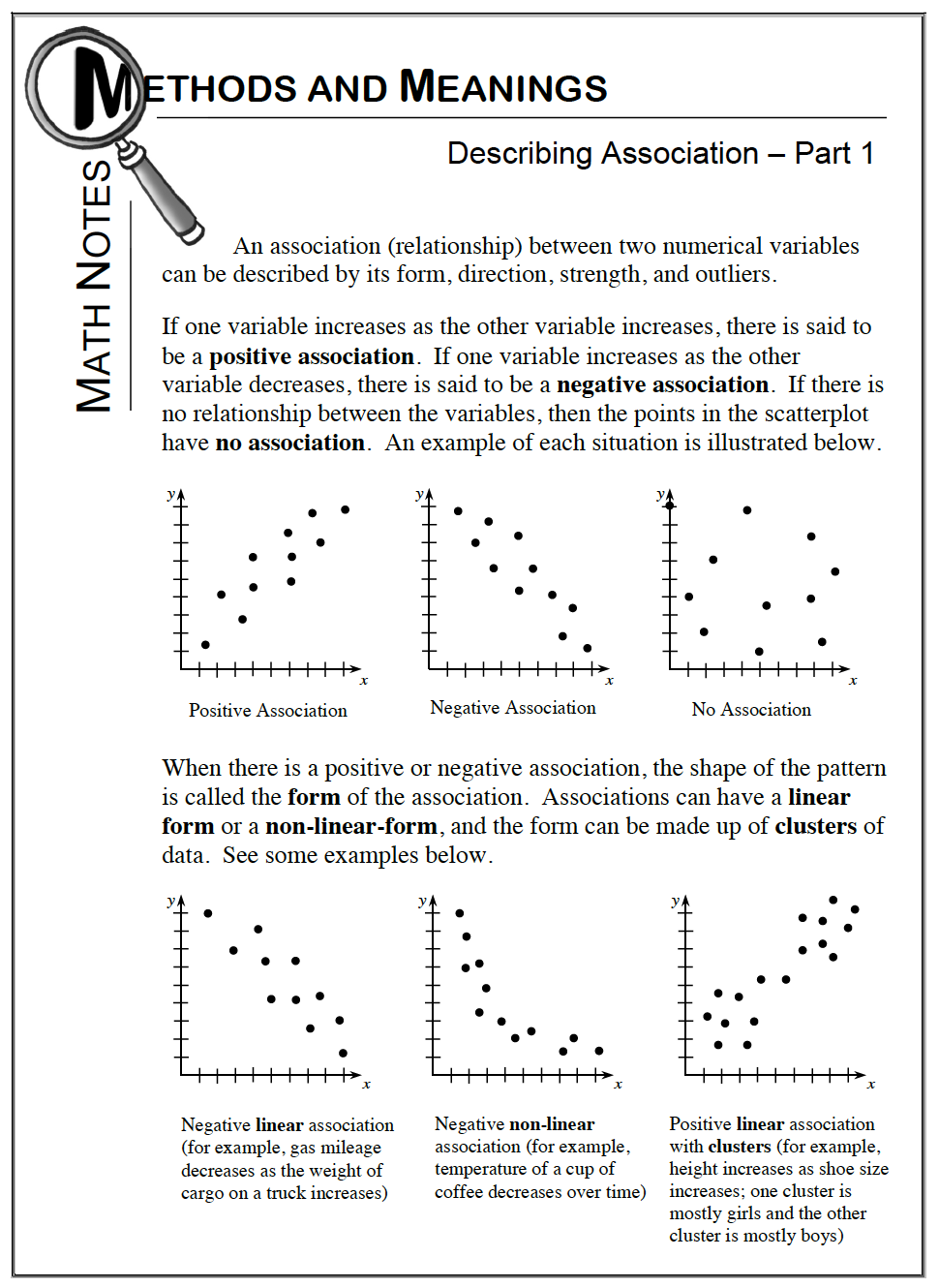### Home > ACC7 > Chapter 7 Unit 8 > Lesson CC3: 7.1.3 > Problem7-29

7-29.

For each scatterplot below, determine if there is an association between the points. Label each graph as showing a positive association, negative association, or no association. If there is an association, write a sentence describing it. Homework Help ✎

Refer to the Math Notes for help.

1.As the number of hours watching TV increases, the grade point average decreases.
The graph has a negative correlation.

1.Positive correlation.

1.Is there any trend in the graph above?